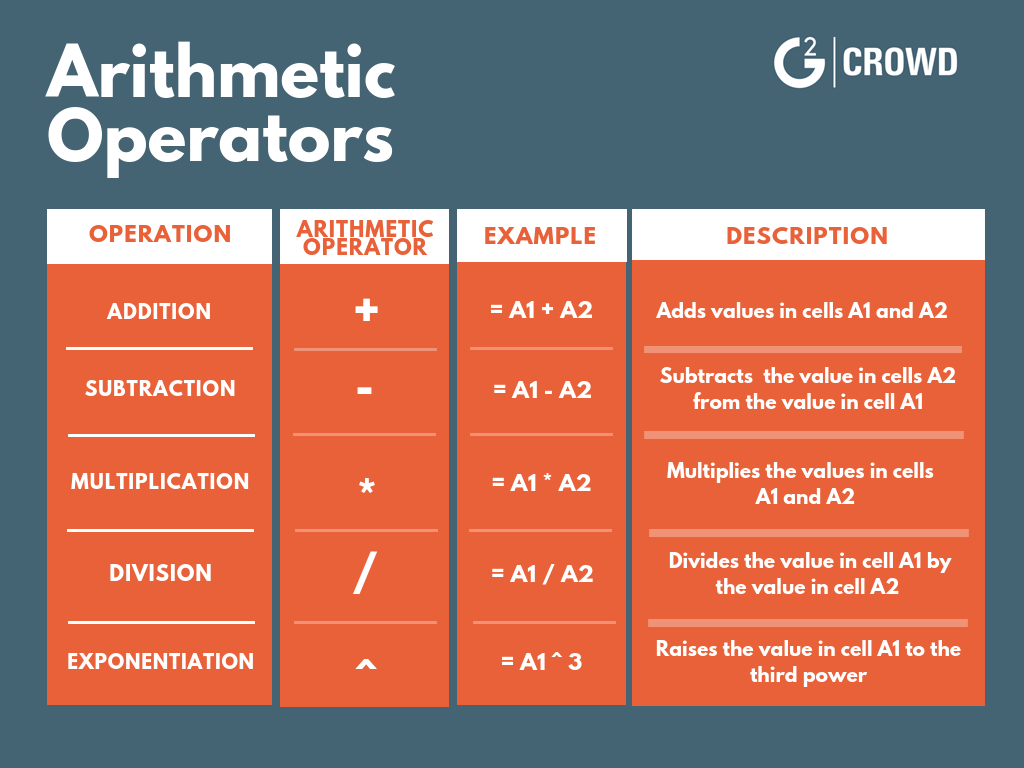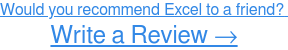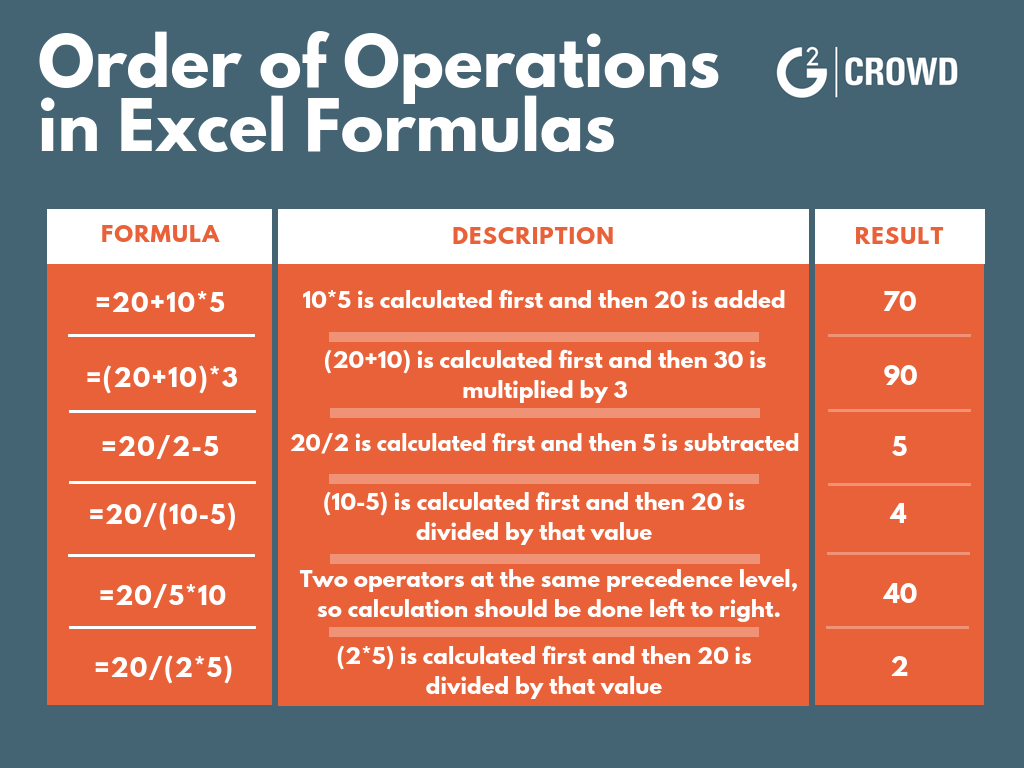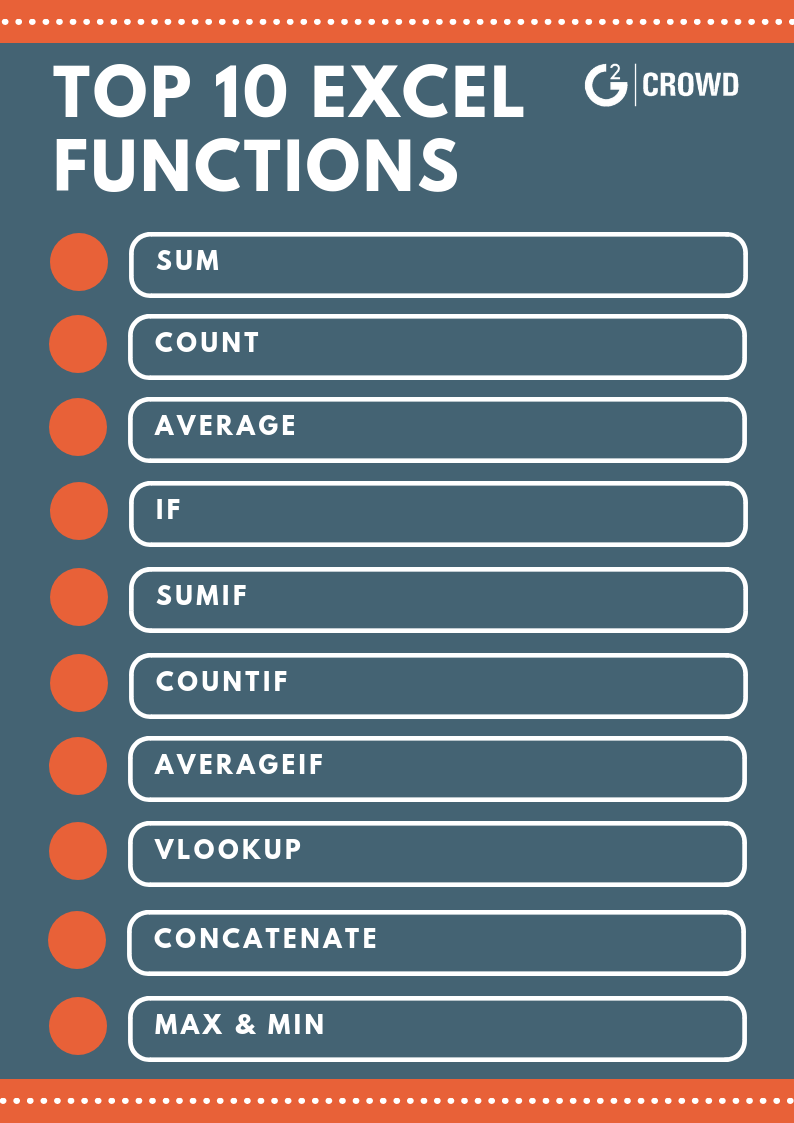# Excel Formulas: How to Enter, Edit, and Simplify (+Tips on Writing Effective Formulas)

Excel can do some crazy-cool things and make your life a whole lot easier once you learn how to use it.

That being said, in this article I’ll be teaching you one of the most basic, and most helpful, features of Microsoft Excel: formulas.

So buckle up, here’s everything you need to know about Excel formulas:

Related:  Want to learn more about specific Excel formulas? Learn how to multiply in Excel and how to merge cells in Excel!

# Excel formulas

Let’s start with defining what an Excel formula is before we jump into the how-tos.

## Entering Excel formulas

All Excel formulas must begin with an equal sign (=) followed by the operation that will be used to return the value. If you don’t start the formula with the equal sign, Excel will assume that you’re entering text, and not a formula.

Formulas are written using arithmetic operators (addition, subtraction, multiplication, etc.) so that the values in the cell are combined to produce a single value as a result.Now that you know about the arithmetic operators within a formula, let’s go over an example of entering an Excel formula.

### Example of an Excel formula

In this example below, we are looking to calculate the charge for the first customer book order. Here are the steps I took to do so:

1. Type your formula, =F13*G13 (the price of the book multiplied by the quantity of books the customer ordered). As you type the formula a list of Excel functions will appear in a pop-up, providing you with a quick method for entering functions (but we’ll cover functions in a different article). Don’t worry, the list will close when you complete the formula.
2. Once you’ve entered the formula, press the ‘Enter’ key. The formula is entered in H13, which will display the value \$25.90 once you’ve pressed ‘Enter’. The result is displayed in currency because cell F13, which is in the formula, contains a currency value.Reminder: Don’t forget that ALL formulas must start with the equal sign for Excel to recognize it as a formula.### Order of operations in Excel

Sometimes your formulas will contain more than one arithmetic operator. In this case, you’ll need to consider the order of operations – the same ol’ order of operations you’ve learned in all of your math classes.

That being said, keep in mind that Excel will first calculate the value of any operation within parenthesis, followed by exponentiation (^), multiplication (*), and division (/), and finally addition (+) and subtraction (-).

Tip: Remember the acronym PEMDAS! Parenthesis, Exponentiation, Multiplication, Division, Addition, Subtraction.## Editing Excel formulas

Let’s face it, we all make mistakes. And sometimes, you might need to go back and edit a formula after initially entering it. Don’t worry, it’s fairly easy!

In this example, I’m going to be editing my original formula from addition to multiplication.

Notice that when I highlight cell A3 my formula populates in the formula bar.To edit this formula, click in the formula bar and edit the operator, changing it from addition to multiplication.Now that you’ve edited what you needed to change, press ‘Enter’!## Copying and pasting Excel formulas

At some point there mere be a case where you’ll need to repeat the same formula throughout your worksheet. Good news – you won’t need to retype the formula over and over, you can copy and paste the formula from one cell to another!

Once you’ve copied a formula, it will be copied to the Excel Clipboard. Then once that formula is pasted, it will be moved from the Clipboard to the selected cell or cell range.

Excel will adjust the cell references in the formula to reflect the formula’s new location. This is because you want to copy the actions of the formula, not the specific value that the formula has generated in the first cell.

Below you’ll find an example of how to copy and paste your Excel formula. By doing this, you’ll save yourself time and avoid potential mistakes that may happen if you had retyped the formula separately.

In this example, we are looking to copy the original formula and paste it into the selected cells. Here are the steps I took to do so:

1. Select cell H13 (the cell containing the original formula that you want to copy).
2. Press Ctrl+C to copy the formula to the clipboard
3. Select the range of cells you want to paste the formula into and press Ctrl+V to paste. Excel will paste the formula into the cells and adjust each formula so that the total charges are calculated based on the corresponding values within each row (since the values in each row vary).Simplifying formulas with Excel functions

While I won’t cover Excel functions in-depth in this article, I will show you where to find the functions to simplify your Excel formulas and the most commonly used functions.

But before I jump into that, what is a function?

Excel’s functions are actually just preset formulas that are available to users.

While functions will auto-populate in a drop-down menu when you start to type your formula in the cell, you can also find the functions categorized at the top of your Excel page (see below).

You can search by clicking 'Insert Function' or browse the lists by clicking on each category separately.There are dozens of useful functions available on Excel – here are the top 10 Excel functions you should start using today:#### SUM function

The SUM function (=SUM) is one of Excel's math and trig functions, and is used to add individual values, cell references, or ranges of cells.

#### COUNT function

The COUNT function is used to calculate the total amount of entries in a defined range that have numbers within the cell.

#### AVERAGE function

The AVERAGE function (=AVERAGE) is used to calculate the average of a selected cell range.

#### IF function

The IF function (=IF) is used to make logical comparisons between values and what you expect.

#### SUMIF function

The SUMIF (=SUMIF) function is used to calculate the sum of values in a range that meet specified criteria.

#### COUNTIF function

The Excel COUNTIF function (=COUNTIF) is one of Excel's statistical functions, and is used to count the number of cells that meet specified criteria.

#### AVERAGEIF function

The AVERAGEIF function (=AVERAGEIF) is used to calculate the average of cells in a range that meet specified criteria.

#### VLOOKUP function

The VLOOKUP function (=VLOOKUP) is used to find values in a table or range by row.

#### CONCATENATE function

The CONCATENATE function (=CONCATENATE) is one of Excel's text functions, and is used to combine two or more strings of text into one string.

#### MAX & MIN functions

The MAX function (=MAX) is used to return the largest value in a set of values. The MIN function (=MIN) is used to return the smallest value in a set of values.

## Tips on writing effective Excel formulas

Excel formulas can make your life a whole lot easier. They can save you time and most of all, a headache from having to do all of the math on your own.

When it comes to writing those formulas, keep these few tips in mind:

### Keep your formulas simple

If possible, use functions in place of those long, complex formulas. Simplifying formulas with functions helps avoid mistakes when entering a formula, ensuring that the formula is making an accurate calculation.

For example, if you’re adding more than two or three cells together, you may consider using the SUM function to make sure you don’t improperly enter a cell and end up with an incorrect value.

### Test your formulas

Before entering the actual values, test your formula with simple values that you can calculate in your head to confirm that your formula is working as expected.

For example, say you're just learning how to subtract in Excel. Use 1’s or 10’s as your input values first – something easy to calculate in your head – to verify your formula was input correctly and is working as intended.

### Don’t hide data values within formulas

The cells will display the result of the formula, not the actual formula.

For example, say you’re looking to calculate a 7 percent interest rate on a value in B5. You could enter the formula =0.07*B5. However, this won’t show how the value is calculated. Instead, place the value 0.07 in a cell accompanied by a label and use the cell reference in the formula. So if you place 0.07 in cell B6, the formula =B6*B5 will calculate the interest value.

This allows others to easily see the interest rate as well as the resulting interest, ensuring that your formula is easy to understand and clearly solving the problem.

## Master your Excel formulas

In this article we’ve covered what Excel formulas are and how to enter, edit, copy and paste, and simplify your Excel formulas – everything you need to know about using Excel formulas!

Now grab your computer and dive into Excel to give it a try for yourself!

Was this article helpful? If so, you can also learn how to make a line graph in Excel  and how to add in Excel in 2018!

Also be sure to check out how project management can help you manage all of your Excel-based projects.

#### Jordan Wahl

Jordan Wahl is a senior content marketing and SEO specialist who joined G2 after graduating with her BBA in Marketing from the University of Wisconsin-Whitewater. She loves anything that puts her in her creative space – including writing, art, and music (her absolute favorite).

News Technology
Finance
Management